Contents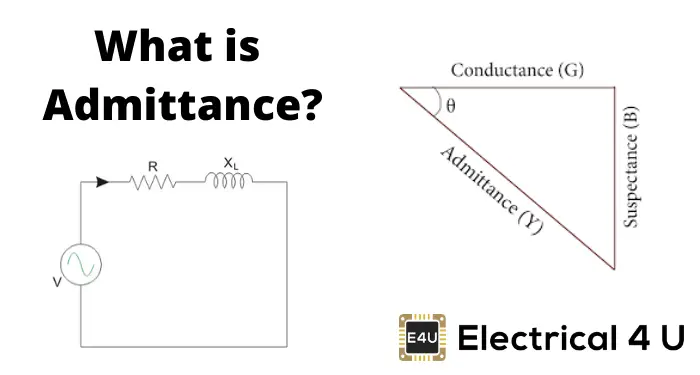Admittance is defined as a measure of how easily a circuit or device will allow current to flow through it. Admittance is the reciprocal (inverse) of impedance, akin to how conductance and resistance are related. The SI unit of admittance is the siemens (symbol S).

To reiterate the above definition: let us first go through some important terms which associated with the topic admittance. We all know that resistance (R) has only magnitude but no phase. We can say that it is the measure of opposition for the flow of current.

In an AC circuit; in addition to the resistance, two impeding mechanisms (inductance and capacitance) have to be considered. So the term impedance is introduced which have the same function of resistance but have both magnitude and phase. Its real part is resistance, and the imaginary part is reactance, which came from the impeding mechanism.

When looking at admittance vs impedance, admittance is the inverse (i.e. the reciprocal) of impedance. Therefore it has the opposite function of impedance. That is, we can say it is the measure of the flow of current which is allowed by a device or a circuit. Admittance also measures the dynamic effects of susceptance of a material to polarization and is measured in Siemens or Mho. Oliver Heaviside introduced this in December 1887.

## Derivation of Admittance from Impedance

Impedance consists of real part (resistance) and imaginary part (reactance). The symbol for impedance is the Z symbol, and the symbol for admittance is the Y symbol.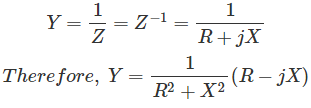Admittance is also a complex number as impedance which is having a real part, Conductance (G) and imaginary part, Susceptance (B).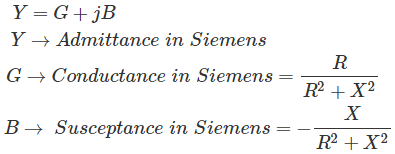(it is negative for capacitive susceptance and positive for inductive susceptance)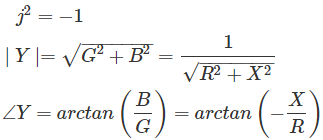It is formed by admittance (Y), susceptance (B) and conductance (G) as shown below.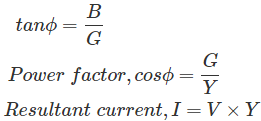## Admittance of a Series Circuit

When a circuit consist of Resistance and Inductance reactance in series is considered as shown below.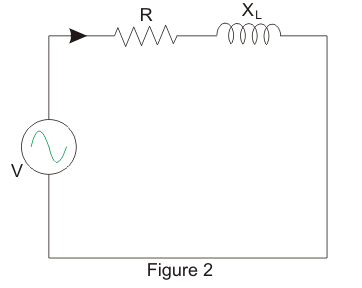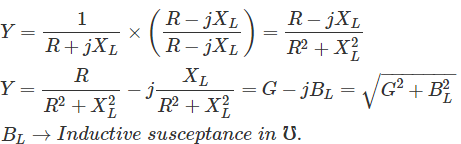When the circuit consist of Resistance and Capacitive reactance in series is considered as shown below.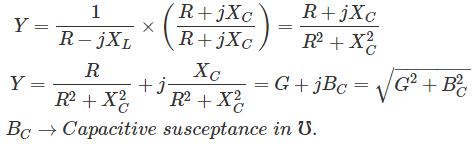## Admittance of a Parallel Circuit

A circuit which consist of two branches say A and B are considered as shown in figure below. ‘A’ comprises of an inductive reactance, XL and a resistance, R1 and ‘B’ comprises of a capacitive reactance, XC and a resistance, R2. The voltage, V is applied to the circuit.For Branch A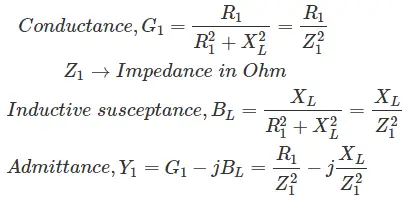For Branch B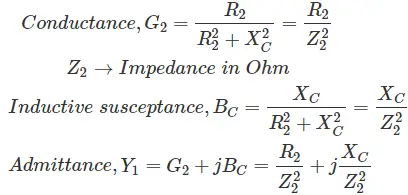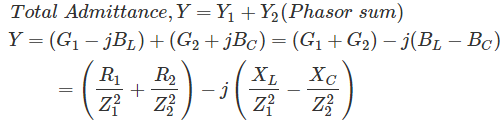So, if the admittance of a circuit is known, then the total current and power factor can be obtained easily.

Want To Learn Faster? 🎓
Get electrical articles delivered to your inbox every week.
No credit card required—it’s 100% free.